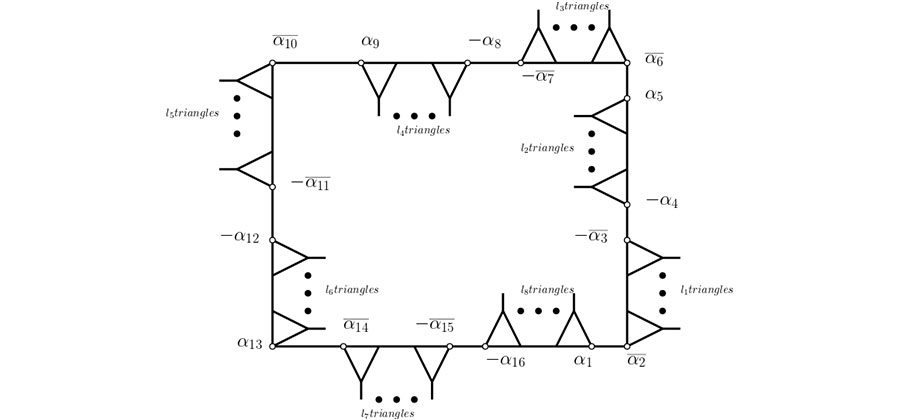• P-ISSN 0974-6846 E-ISSN 0974-5645# Indian Journal of Science and Technology

## Article• VIEWS 937
• PDF 252

Indian Journal of Science and Technology

Year: 2020, Volume: 13, Issue: 22, Pages: 2220-2228

Original Article

## On circuit structure of PSL (2, Z)-orbits of length eight

Received Date:30 May 2020, Accepted Date:12 June 2020, Published Date:25 June 2020

## Abstract

Background/Objectives: Modular group or PSL(2,Z) is a well-known group of the non-singular square matrices of order two by two with unit determinant. An Action of this group on real quadratic fields is represented by coset diagrams consisting of closed paths known as circuits. For a particular value of n , one or more than one circuits combine to form an orbit. The length of a circuit identifies all the circuits of an orbit. The main objective of this study is to explore all the circuits of length eight and their corresponding orbits. Methods: Some circuit equivalent properties, the group theoretical approach, along with the statistical methodology, is adopted to classify the orbits containing circuits of length eight. We also use already discovered results related to circuits of lengths two, four, and six to formulate the basis for our new results of length eight. Findings: We have discovered all the equivalence classes for the circuits of length eight, which are twenty-one in number. For a particular reduced number α, circuits of length eight can have all four; α,−α, its algebraic conjugateα¯ and − α¯ , either in one circuit or (α) G = (−α) G with (−α) G = (−α¯) G or (α) G = (α¯) G with (−α) G = (−α) G or (α) G = (−α) G with (−α) G = (α) G , depending upon the equivalent class of the circuit. Moreover, we have introduced reduced positions and G-midway and discovered that for any reduced numbers αi starting from α1 , reduced positions have a recurring pattern α2,−α3,−α4,α5, and so on. Applications: PSL(2, Z) orbits are entirely classified and drawn, along with the cyclically equivalent circuits of length eight.

Keywords: Modular group; coset diagram; reduced numbers; G-midway; reduced positions

## References

1. Cayley A. The theory of groups, graphical representations. American Journal of Mathematics. 1878;p. 174–176.
2. Higman G, Mushtaq Q. Coset diagrams and relations for PSL(2, Z) Arab Gulf Journal of Scientific Research. 1983;1(1):159–164.
3. Mushtaq Q. Modular group acting on real quadratic fields. Bulletin of the Australian Mathematical Society. 1988;37(2):303–309. Available from: https://dx.doi.org/10.1017/s000497270002685x
4. Malik MA, Husnine SM, Majeed A. Modular Group Action on Certain Quadratic Fields. Punjab University Journal of Mathematics. 1995;28:47–68.
5. Malik MA, SA. Reduced quadratic irrational numbers and types of G-circuits with length four by modular group. Indian Journal of Science and technology. 2018;11:1–7.
6. Ali S, Ma M. Classification of PSL(2, Z) circuits having length six. Indian Journal of Science and technology. 2018;11(42):1–18.
7. Mushtaq Q, Razaq A, Yousaf A. On contraction of vertices of the circuits in coset diagrams for PSL(2, Z) Proceedings Mathematical Sciences. 2018;129(1).
8. Yousaf A, Alolaiyan H, Razaq A, Younis M. Evolution of ambiguous numbers under the actions of a Bianchi group. Journal of Taibah University for Science. 2020;14(1):615–620. Available from: https://dx.doi.org/10.1080/16583655.2020.1760511
9. Razaq A, Mushtaq Q, Yousaf A. The number of circuits of length 4 in PSL(2,ℤ)-space. Communications in Algebra. 2018;46(12):5136–5145. Available from: https://dx.doi.org/10.1080/00927872.2018.1461880
10. Mushtaq Q, Razaq A. Partial proof of Graham Higman's conjecture related to coset diagrams. Bulletin of the Iranian Mathematical Society. 2016;42(2):353–369.
11. Ascioglu M, Cangul IN. Narumi–Katayama index of the subdivision graphs. Journal of Taibah University for Science. 2018;12(4):401–408. Available from: https://dx.doi.org/10.1080/16583655.2018.1474542
12. Gunes AY, Togan M, Celik F, Cangul IN. Cut vertex and cut edge problem for topological graph indices. Journal of Taibah University for Science. 2019;13(1):1175–1183. Available from: https://dx.doi.org/10.1080/16583655.2019.1695520
13. Javed MA, Malik MA. Properties of circuits in coset diagrams by modular group. Indian Journal of Science and Technology.2020. 13(14):1–12.
14. M, Malik A, Riaz M. G-subsets and G-orbits of Q(sqrt n) under action of the Modular Group. Punjab University Journal of Mathematics. 2011;43:75–84.
15. Malik MA, MR. ORBITS OF Q′ ∗ ( √ k 2m) UNDER THE ACTION OF THE MODULAR GROUP P SL(2, Z) University Politehnica of Bucharest Scientific Bulletin-Series A-Applied Mathematics and Physics. 2012;74(4):109–116.Article Metrics